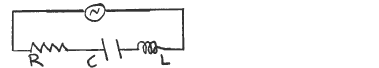# Problem: In an R-L-C ac series circuit the voltage across the capacitor is 64 V and the voltage amplitude across the inductor is 96 V. Does the source voltage lag or lead the current in the circuit?(a) lag(b) lead

###### Problem Details

In an R-L-C ac series circuit the voltage across the capacitor is 64 V and the voltage amplitude across the inductor is 96 V. Does the source voltage lag or lead the current in the circuit?

(a) lag